# 2.7 Linear inequalities and absolute value inequalities  (Page 5/11)

 Page 5 / 11

## Verbal

When solving an inequality, explain what happened from Step 1 to Step 2:

When we divide both sides by a negative it changes the sign of both sides so the sense of the inequality sign changes.

When solving an inequality, we arrive at:

$\begin{array}{l}x+2

Explain what our solution set is.

When writing our solution in interval notation, how do we represent all the real numbers?

$\left(-\infty ,\infty \right)$

When solving an inequality, we arrive at:

$\begin{array}{l}x+2>x+3\hfill \\ \phantom{\rule{1.2em}{0ex}}2>3\hfill \end{array}$

Explain what our solution set is.

Describe how to graph $\text{\hspace{0.17em}}y=|x-3|$

We start by finding the x -intercept, or where the function = 0. Once we have that point, which is $\text{\hspace{0.17em}}\left(3,0\right),$ we graph to the right the straight line graph $\text{\hspace{0.17em}}y=x-3,$ and then when we draw it to the left we plot positive y values, taking the absolute value of them.

## Algebraic

For the following exercises, solve the inequality. Write your final answer in interval notation.

$4x-7\le 9$

$3x+2\ge 7x-1$

$\left(-\infty ,\frac{3}{4}\right]$

$-2x+3>x-5$

$4\left(x+3\right)\ge 2x-1$

$\left[\frac{-13}{2},\infty \right)$

$-\frac{1}{2}x\le \frac{-5}{4}+\frac{2}{5}x$

$-5\left(x-1\right)+3>3x-4-4x$

$\left(-\infty ,3\right)$

$-3\left(2x+1\right)>-2\left(x+4\right)$

$\frac{x+3}{8}-\frac{x+5}{5}\ge \frac{3}{10}$

$\left(-\infty ,-\frac{37}{3}\right]$

$\frac{x-1}{3}+\frac{x+2}{5}\le \frac{3}{5}$

For the following exercises, solve the inequality involving absolute value. Write your final answer in interval notation.

$|x+9|\ge -6$

All real numbers $\text{\hspace{0.17em}}\left(-\infty ,\infty \right)$

$|2x+3|<7$

$|3x-1|>11$

$\left(-\infty ,\frac{-10}{3}\right)\cup \left(4,\infty \right)$

$|2x+1|+1\le 6$

$|x-2|+4\ge 10$

$\left(-\infty ,-4\right]\cup \left[8,+\infty \right)$

$|-2x+7|\le 13$

$|x-7|<-4$

No solution

$|x-20|>-1$

$|\frac{x-3}{4}|<2$

$\left(-5,11\right)$

For the following exercises, describe all the x -values within or including a distance of the given values.

Distance of 5 units from the number 7

Distance of 3 units from the number 9

$\left[6,12\right]$

Distance of10 units from the number 4

Distance of 11 units from the number 1

$\left[-10,12\right]$

For the following exercises, solve the compound inequality. Express your answer using inequality signs, and then write your answer using interval notation.

$-4<3x+2\le 18$

$3x+1>2x-5>x-7$

$3y<5-2y<7+y$

$x+7

## Graphical

For the following exercises, graph the function. Observe the points of intersection and shade the x -axis representing the solution set to the inequality. Show your graph and write your final answer in interval notation.

$|x-1|>2$

$\left(-\infty ,-1\right)\cup \left(3,\infty \right)$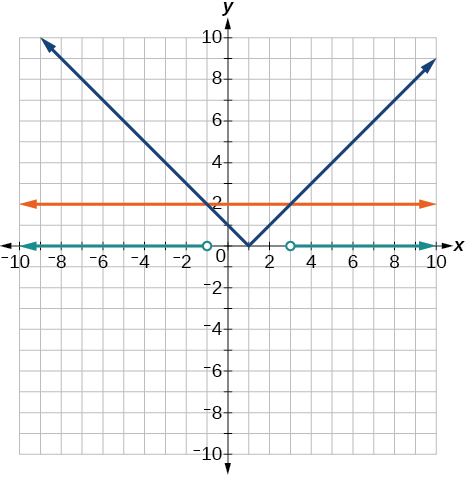$|x+3|\ge 5$

$|x+7|\le 4$

$\left[-11,-3\right]$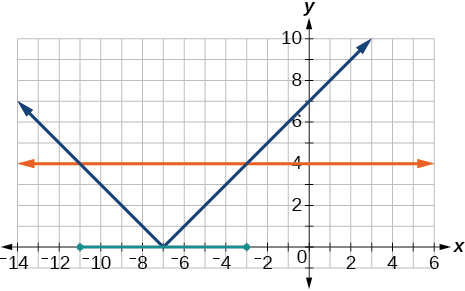$|x-2|<7$

$|x-2|<0$

It is never less than zero. No solution.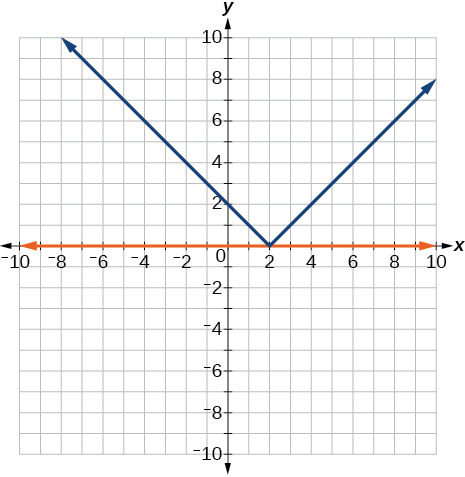For the following exercises, graph both straight lines (left-hand side being y1 and right-hand side being y2) on the same axes. Find the point of intersection and solve the inequality by observing where it is true comparing the y -values of the lines.

$x+3<3x-4$

$x-2>2x+1$

Where the blue line is above the orange line; point of intersection is $\text{\hspace{0.17em}}x=-3.$

$\left(-\infty ,-3\right)$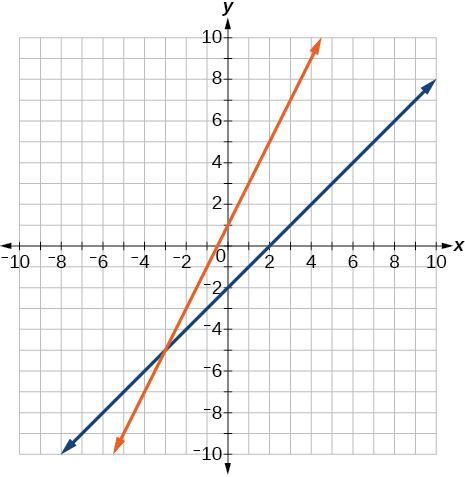$x+1>x+4$

$\frac{1}{2}x+1>\frac{1}{2}x-5$

Where the blue line is above the orange line; always. All real numbers.

$\left(-\infty ,-\infty \right)$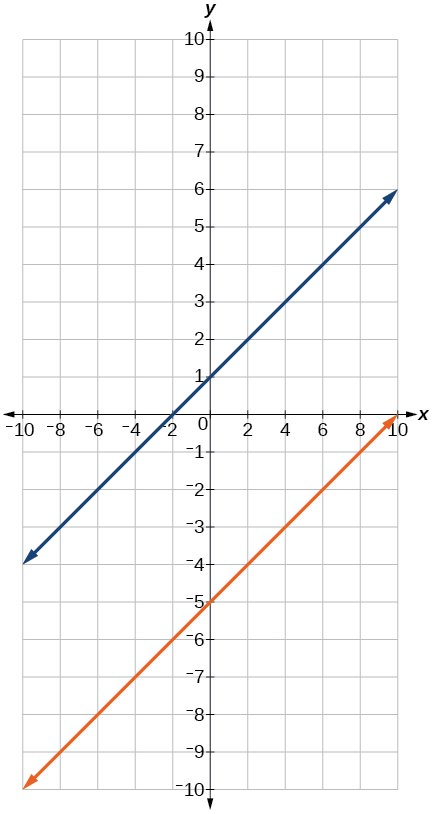$4x+1<\frac{1}{2}x+3$

## Numeric

For the following exercises, write the set in interval notation.

$\left\{x|-1

$\left(-1,3\right)$

$\left\{x|x\ge 7\right\}$

$\left\{x|x<4\right\}$

$\left(-\infty ,4\right)$

For the following exercises, write the interval in set-builder notation.

$\left(-\infty ,6\right)$

$\left\{x|x<6\right\}$

$\left(4,+\infty \right)$

$\left[-3,5\right)$

$\left\{x|-3\le x<5\right\}$

$\left[-4,1\right]\cup \left[9,\infty \right)$

For the following exercises, write the set of numbers represented on the number line in interval notation.

#### Questions & Answers

if sin15°=√p, express the following in terms of p
Norman Reply
prove sin²x+cos²x=3+cos4x
Kiddy Reply
the difference between two signed numbers is -8.if the minued is 5,what is the subtrahend
jeramie Reply
the difference between two signed numbers is -8.if the minuend is 5.what is the subtrahend
jeramie
what are odd numbers
micheal Reply
numbers that leave a remainder when divided by 2
Thorben
1,3,5,7,... 99,...867
Thorben
7%2=1, 679%2=1, 866245%2=1
Thorben
the third and the seventh terms of a G.P are 81 and 16, find the first and fifth terms.
Suleiman Reply
if a=3, b =4 and c=5 find the six trigonometric value sin
Martin Reply
ask
Ans
pls how do I factorize x⁴+x³-7x²-x+6=0
Gift Reply
in a function the input value is called
Rimsha Reply
how do I test for values on the number line
Modesta Reply
if a=4 b=4 then a+b=
Rimsha Reply
a+b+2ab
Kin
commulative principle
DIOSDADO
a+b= 4+4=8
Mimi
If a=4 and b=4 then we add the value of a and b i.e a+b=4+4=8.
Tariq
what are examples of natural number
sani Reply
an equation for the line that goes through the point (-1,12) and has a slope of 2,3
Katheryn Reply
3y=-9x+25
Ishaq
show that the set of natural numberdoes not from agroup with addition or multiplication butit forms aseni group with respect toaaddition as well as multiplication
Komal Reply
x^20+x^15+x^10+x^5/x^2+1
Urmila Reply
evaluate each algebraic expression. 2x+×_2 if ×=5
Sarch Reply

### Read also:

#### Get Jobilize Job Search Mobile App in your pocket Now!

Source:  OpenStax, Algebra and trigonometry. OpenStax CNX. Nov 14, 2016 Download for free at https://legacy.cnx.org/content/col11758/1.6
Google Play and the Google Play logo are trademarks of Google Inc.

Notification Switch

Would you like to follow the 'Algebra and trigonometry' conversation and receive update notifications?ByByBy Olivia D'AmbrogioBy Sandhills MLTBy Brooke DelaneyBy Anonymous UserBy OpenStaxBy OpenStaxBy Jonathan LongBy OpenStaxBy OpenStaxBy Rohini Ajay